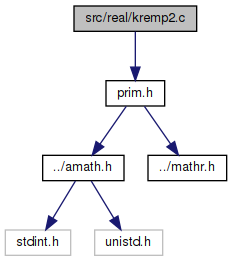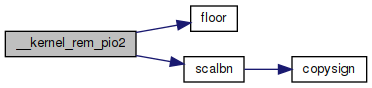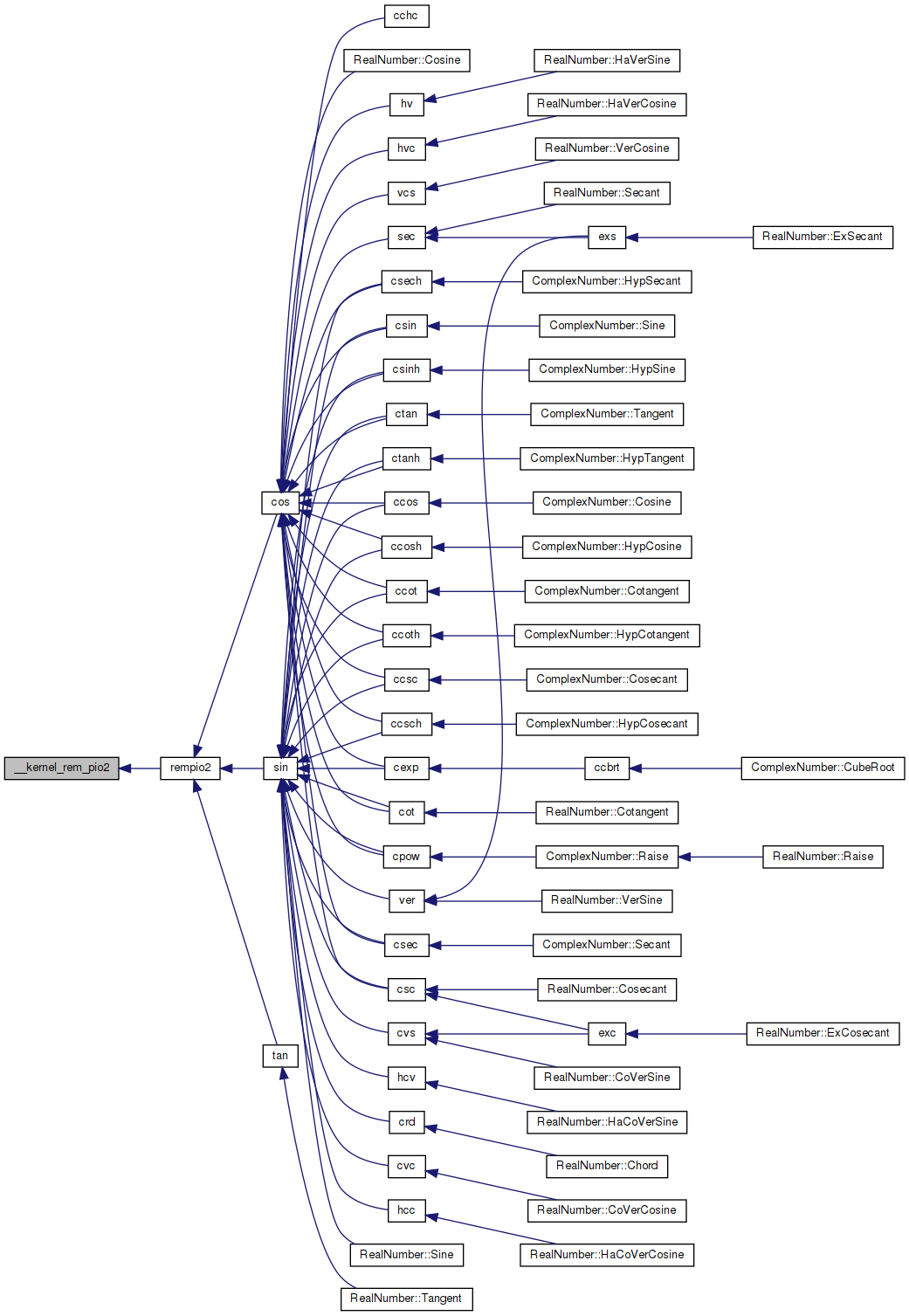amath  1.8.5 Simple command line calculator
kremp2.c File Reference

Kernel reduction function. More...

`#include "prim.h"`
Include dependency graph for kremp2.c:Go to the source code of this file.

## Functions

int __kernel_rem_pio2 (double *x, double *y, int e0, int nx, int prec, const int *ipio2)
Kernel reduction function. More...

## Variables

static const int init_jk [] = {2,3,4,6}

static const double PIo2 []

static const double zero = 0.0

static const double one = 1.0

static const double two24 = 1.67772160000000000000e+07

static const double twon24 = 5.96046447753906250000e-08

## Detailed Description

Kernel reduction function.

Definition in file kremp2.c.

## ◆ __kernel_rem_pio2()

 int __kernel_rem_pio2 ( double * x, double * y, int e0, int nx, int prec, const int * ipio2 )

Kernel reduction function.

```__kernel_rem_pio2(x,y,e0,nx,prec,ipio2)
double x[],y[]; int e0,nx,prec; int ipio2[];```
```__kernel_rem_pio2 return the last three digits of N with
y = x - N*pi/2
so that |y| < pi/2.```
```The method is to compute the integer (mod 8) and fraction parts of
(2/pi)*x without doing the full multiplication. In general we
skip the part of the product that are known to be a huge integer (
more accurately, = 0 mod 8 ). Thus the number of operations are
independent of the exponent of the input.```
`(2/pi) is represented by an array of 24-bit integers in ipio2[].`
```Input parameters:
x[] The input value (must be positive) is broken into nx
pieces of 24-bit integers in double precision format.
x[i] will be the i-th 24 bit of x. The scaled exponent
of x is given in input parameter e0 (i.e., x*2^e0
match x's up to 24 bits.```
```    Example of breaking a double positive z into x+x+x:
e0 = ilogb(z)-23
z  = scalbn(z,-e0)
for i = 0,1,2
x[i] = floor(z)
z    = (z-x[i])*2**24```
``` y[]    ouput result in an array of double precision numbers.
The dimension of y[] is:
24-bit  precision   1
53-bit  precision   2
64-bit  precision   2
113-bit precision   3
The actual value is the sum of them. Thus for 113-bit
precison, one may have to do something like:```
```    long double t,w,r_head, r_tail;
t = (long double)y + (long double)y;
w = (long double)y;
r_tail = w - (r_head - t);```
` e0 The exponent of x`
` nx dimension of x[]`
```    prec    an integer indicating the precision:
0   24  bits (single)
1   53  bits (double)
2   64  bits (extended)
``` ipio2[]
integer array, contains the (24*i)-th to (24*i+23)-th
bit of 2/pi after binary point. The corresponding
floating value is
ipio2[i] * 2^(-24(i+1)).
```
```External function:
double scalbn(), floor();```
```Here is the description of some local variables:
jk  jk+1 is the initial number of terms of ipio2[] needed
in the computation. The recommended value is 2,3,4,
6 for single, double, extended,and quad.

jz  local integer variable indicating the number of
terms of ipio2[] used.
```
` jx nx - 1`
``` jv index for pointing to the suitable ipio2[] for the
computation. In general, we want
( 2^e0*x * ipio2[jv-1]*2^(-24jv) )/8
is an integer. Thus
e0-3-24*jv >= 0 or (e0-3)/24 >= jv
Hence jv = max(0,(e0-3)/24).```
` jp jp+1 is the number of terms in PIo2[] needed, jp = jk.`
```    q[] double array with integral value, representing the
24-bits chunk of the product of x and 2/pi.```
``` q0 the corresponding exponent of q. Note that the
exponent for q[i] would be q0-24*i.```
``` PIo2[] double precision array, obtained by cutting pi/2
into 24 bits chunks.```
` f[]    ipio2[] in floating point`
` iq[]   integer array by breaking up q[] in 24-bits chunk.`
` fq[]   final product of x*(2/pi) in fq,..,fq[jk]`
``` ih integer. If >0 it indicates q[] is >= 0.5, hence
it also indicates the sign of the result.
```

Definition at line 184 of file kremp2.c.

References floor(), init_jk, one, PIo2, scalbn(), two24, twon24, and zero.

Referenced by rempio2().

185 {
186  int jz,jx,jv,jp,jk,carry,n,iq,i,j,k,m,q0,ih;
187  double z,fw,f,fq,q;
188
189  /* initialize jk*/
190  jk = init_jk[prec];
191  jp = jk;
192
193  /* determine jx,jv,q0, note that 3>q0 */
194  jx = nx-1;
195  jv = (e0-3)/24;
196  if(jv<0) jv=0;
197  q0 = e0-24*(jv+1);
198
199  /* set up f to f[jx+jk] where f[jx+jk] = ipio2[jv+jk] */
200  j = jv-jx;
201  m = jx+jk;
202  for(i=0; i<=m; i++,j++) f[i] = (j<0)? zero : (double) ipio2[j];
203
204  /* compute q,q,...q[jk] */
205  for (i=0; i<=jk; i++) {
206  for(j=0,fw=0.0; j<=jx; j++) fw += x[j]*f[jx+i-j];
207  q[i] = fw;
208  }
209
210  jz = jk;
211 recompute:
212  /* distill q[] into iq[] reversingly */
213  for(i=0,j=jz,z=q[jz]; j>0; i++,j--) {
214  fw = (double)((int)(twon24* z));
215  iq[i] = (int)(z-two24*fw);
216  z = q[j-1]+fw;
217  }
218
219  /* compute n */
220  z = scalbn(z,q0); /* actual value of z */
221  z -= 8.0*floor(z*0.125); /* trim off integer >= 8 */
222  n = (int) z;
223  z -= (double)n;
224  ih = 0;
225  if(q0>0) { /* need iq[jz-1] to determine n */
226  i = (iq[jz-1]>>(24-q0));
227  n += i;
228  iq[jz-1] -= i<<(24-q0);
229  ih = iq[jz-1]>>(23-q0);
230  }
231  else if(q0==0) ih = iq[jz-1]>>23;
232  else if(z>=0.5) ih=2;
233
234  if(ih>0) { /* q > 0.5 */
235  n += 1;
236  carry = 0;
237  for(i=0; i<jz ; i++) { /* compute 1-q */
238  j = iq[i];
239  if(carry==0) {
240  if(j!=0) {
241  carry = 1;
242  iq[i] = 0x1000000- j;
243  }
244  } else iq[i] = 0xffffff - j;
245  }
246  if(q0>0) { /* rare case: chance is 1 in 12 */
247  switch(q0) {
248  case 1:
249  iq[jz-1] &= 0x7fffff;
250  break;
251  case 2:
252  iq[jz-1] &= 0x3fffff;
253  break;
254  }
255  }
256  if(ih==2) {
257  z = one - z;
258  if(carry!=0) z -= scalbn(one,q0);
259  }
260  }
261
262  /* check if recomputation is needed */
263  if(z==zero) {
264  j = 0;
265  for (i=jz-1; i>=jk; i--) j |= iq[i];
266  if(j==0) { /* need recomputation */
267  for(k=1; iq[jk-k]==0; k++); /* k = no. of terms needed */
268
269  for(i=jz+1; i<=jz+k; i++) { /* add q[jz+1] to q[jz+k] */
270  f[jx+i] = (double) ipio2[jv+i];
271  for(j=0,fw=0.0; j<=jx; j++) fw += x[j]*f[jx+i-j];
272  q[i] = fw;
273  }
274  jz += k;
275  goto recompute;
276  }
277  }
278
279  /* chop off zero terms */
280  if(z==0.0) {
281  jz -= 1;
282  q0 -= 24;
283  while(iq[jz]==0) {
284  jz--;
285  q0-=24;
286  }
287  } else { /* break z into 24-bit if necessary */
288  z = scalbn(z,-q0);
289  if(z>=two24) {
290  fw = (double)((int)(twon24*z));
291  iq[jz] = (int)(z-two24*fw);
292  jz += 1;
293  q0 += 24;
294  iq[jz] = (int) fw;
295  } else iq[jz] = (int) z ;
296  }
297
298  /* convert integer "bit" chunk to floating-point value */
299  fw = scalbn(one,q0);
300  for(i=jz; i>=0; i--) {
301  q[i] = fw*(double)iq[i];
302  fw*=twon24;
303  }
304
305  /* compute PIo2[0,...,jp]*q[jz,...,0] */
306  for(i=jz; i>=0; i--) {
307  for(fw=0.0,k=0; k<=jp&&k<=jz-i; k++) fw += PIo2[k]*q[i+k];
308  fq[jz-i] = fw;
309  }
310
311  /* compress fq[] into y[] */
312  switch(prec) {
313  case 0:
314  fw = 0.0;
315  for (i=jz; i>=0; i--) fw += fq[i];
316  y = (ih==0)? fw: -fw;
317  break;
318  case 1:
319  case 2:
320  fw = 0.0;
321  for (i=jz; i>=0; i--) fw += fq[i];
322  y = (ih==0)? fw: -fw;
323  fw = fq-fw;
324  for (i=1; i<=jz; i++) fw += fq[i];
325  y = (ih==0)? fw: -fw;
326  break;
327  case 3: /* painful */
328  for (i=jz; i>0; i--) {
329  fw = fq[i-1]+fq[i];
330  fq[i] += fq[i-1]-fw;
331  fq[i-1] = fw;
332  }
333  for (i=jz; i>1; i--) {
334  fw = fq[i-1]+fq[i];
335  fq[i] += fq[i-1]-fw;
336  fq[i-1] = fw;
337  }
338  for (fw=0.0,i=jz; i>=2; i--) fw += fq[i];
339  if(ih==0) {
340  y = fq;
341  y = fq;
342  y = fw;
343  } else {
344  y = -fq;
345  y = -fq;
346  y = -fw;
347  }
348  }
349  return n&7;
350 }
static const double two24
Definition: kremp2.c:72
static const double twon24
Definition: kremp2.c:73
double scalbn(double x, int n)
static const int init_jk[]
Definition: kremp2.c:56
static const double PIo2[]
Definition: kremp2.c:58
static const double zero
Definition: kremp2.c:70
static const double one
Definition: kremp2.c:71
double floor(double x)
Floor function.
Definition: floor.c:62
Here is the call graph for this function:Here is the caller graph for this function:## ◆ init_jk

 const int init_jk[] = {2,3,4,6}
static

Definition at line 56 of file kremp2.c.

Referenced by __kernel_rem_pio2().

## ◆ one

 const double one = 1.0
static

Definition at line 71 of file kremp2.c.

Referenced by __kernel_rem_pio2().

## ◆ PIo2

 const double PIo2[]
static
Initial value:
= {
1.57079625129699707031e+00,
7.54978941586159635335e-08,
5.39030252995776476554e-15,
3.28200341580791294123e-22,
1.27065575308067607349e-29,
1.22933308981111328932e-36,
2.73370053816464559624e-44,
2.16741683877804819444e-51,
}

Definition at line 58 of file kremp2.c.

Referenced by __kernel_rem_pio2().

## ◆ two24

 const double two24 = 1.67772160000000000000e+07
static

Definition at line 72 of file kremp2.c.

Referenced by __kernel_rem_pio2().

## ◆ twon24

 const double twon24 = 5.96046447753906250000e-08
static

Definition at line 73 of file kremp2.c.

Referenced by __kernel_rem_pio2().

## ◆ zero

 const double zero = 0.0
static

Definition at line 70 of file kremp2.c.

Referenced by __kernel_rem_pio2().